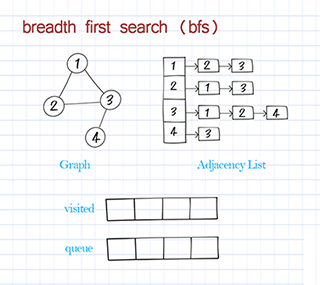A graph is a data structure that consists of a set of  nodes connected by edges. Graphs are used to simulate many real-world problems, such as paths in cities, circuit networks, and social networks. This is graph implementation part 1 – unweighted graph.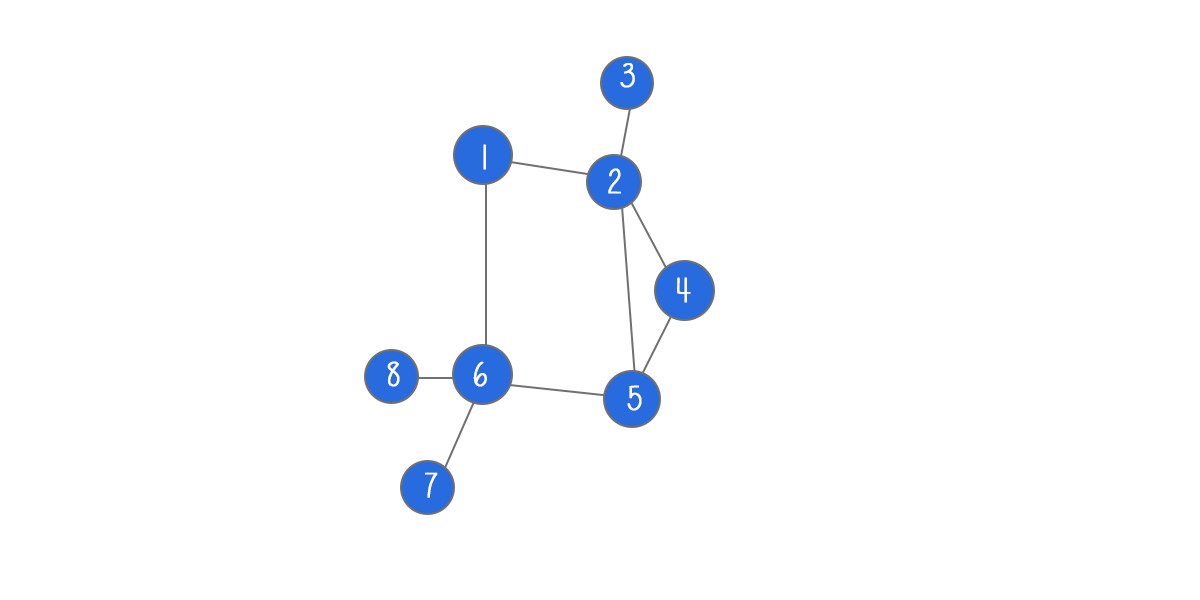### How to represent nodes and edges in adjacency list?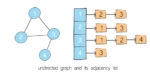An adjacency list is a list of list: a list of nodes, with each node has a list of neighbors. The underneath data structure is a hash map. The buckets of hash map hold all nodes(vertices) in a graph. The node is the key. The value is the linked list of the node’s neighbors.

### Terminology

A node in the graph is also called vertex.  A line between two nodes is edge. Two nodes are adjacent (or neighbors) if they are connected to each other through an edge. Path represents a sequence of edges between the two nodes.

In a directed graph, all of the edges represent a one-way relationship. In an undirected graph, all edges are bi-directional.

If the edges in the graph have weights (represent costs or distances), the graph is said to be a weighted graph. If the edges do not have weights, the graph is said to be unweighted.

Graph can be presented as adjacency list or adjacency matrix. An adjacency list is an array of edges or nodes. Adjacency list is used for representation of the sparse graphs and used more often. An adjacency matrix is a square matrix with dimensions equivalent to the number of nodes in the graph. Adjacency matrix is preferred when the graph is dense.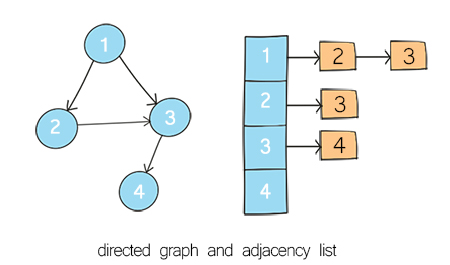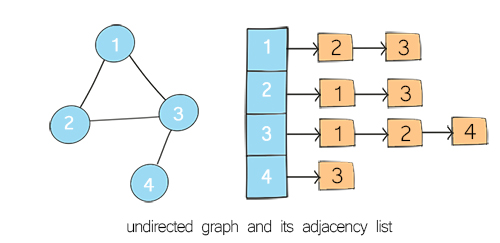### You are herePart 1 – Graph implementation as adjacency list
Part 2 – Weighted graph as adjacency list
Part 3 – Graph as adjacency matrix

The Graph class has two fields: adj and directedadj is a HashMap in which the key is the node, the value is all its neighbors. The value is represented as linked list of the nodes. directed is a boolean variable to specify whether the graph is directed or undirected. By default, it is undirected.

In many old implementation using adjacency list, adj is defined as an array of node list. The disadvantage of this implementation is that we have to define the length of the array first. To avoid this, we use hashmap. By using hashmap, we can retrieve node and its neighbors by node itself, not by index in array. Note the node can be any data type, for example primitive data type, such as integer or string, or an object, such as graphNode.

To add a node to the graph is to add a key in the hashmap. To add an edge is to add an item in this key’s value. The following method addEdge includes both adding a node and adding an edge. For a directed graph, we add edge from a to b. For undirected graph, we also add edge from b to a.

## Doodle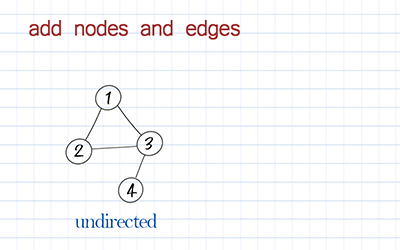### Remove node and edge

Remove operation includes remove edge and remove node. To remove edge, we use the node as key to find its neighbors in the hashmap. Then remove the other node from its neighbors. For a directed graph, we just need to remove edge from a to b. For an undirected graph, we also need to remove the edge from b to a.

## Doodle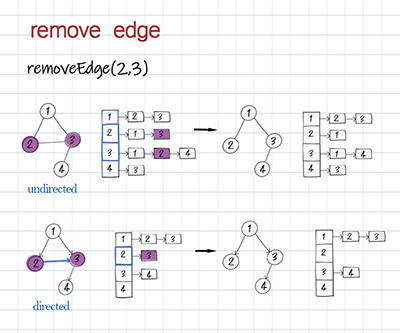Remove node has more work to do than remove edge. We have to remove all connected edge before remove the node itself. For an undirected graph, first we get all neighbors of the node. Then for each of its neighbors, remove itself from the value list. For a directed graph, we search all keys in the hashmap for their values, and check whether this node exists in their neighbors. If it does, remove it. The last step is to remove the node as the key in the hashmap. Then this node is no longer in the hashmap’s key set.

## Doodle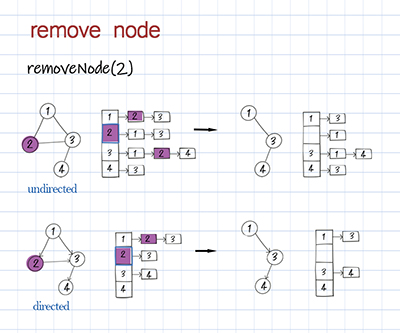Search can be search node, edge or path. We can check whether there is a node existing in the graph. This can be done by simply checking the hashmap contains the key. We can also check whether there is a direct connection between two nodes (aka whether there is an edge). This can be done by checking whether the other node is in one node’s neighbors.

## Doodle### Find path with depth first search in graph

More useful operation is to search path. A path is a sequence of edges. There can be more than one path between two nodes. There are two common approaches: depth first search (DFS) and breadth first search (BFS). In this section, we use DFS and BFS to find out whether there is path from one node to another. In traversal section, we use them to find all reachable nodes from the source node in graph. DFS and BFS is most importation part of graph implementation.

Depth First Search starts from the source node, and explores the adjacent nodes as far as possible before call back. DFS is usually implemented with recursion or stack. It is used to solve find path or detect cycle problems.

## Doodle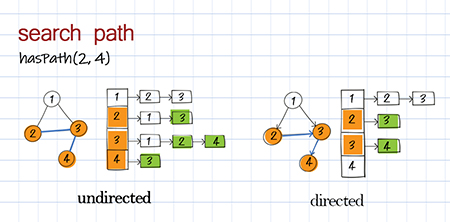### Find path with breadth first search in graph

Breath First Search starts from the source node, and explores all its adjacent nodes before going to the next level adjacent nodes. BFS is usually implemented with Queue. It is often used to solve shortest path problems.

## Python

### Print graph as adjacency list

Print is to visit all nodes in the graph and print the information stored. This is actually to the hashmap. It can be done by looping through the key set of the hashmap. This method is used for debugging purpose.

## Python

### Traverse with depth first search in graph

DFS traversal: Use depth first search to visit nodes in the graph and print the node’s information. This is similar to DFS traversal in binary tree. Starting from the source node, we call recursive method to visit its neighbor’s neighbor until call back. Please node the source might be any node in the graph. Some nodes might not be reached in a directed graph. (In binary tree, we always start from the root and all nodes should be visited.)

## Doodle### Traverse with breadth first search in graph

BFS traversal: Use breadth first search to visit all nodes in the graph and print the node’s information. This is similar to BFS traversal in binary tree. Starting from the source, visit all its neighbors first before visiting neighbor’s neighbor. Please node the source might be any node in the graph. Some nodes might not be reached in a directed graph. (In binary tree, we always start from the root and all nodes should be visited.)

## Doodle